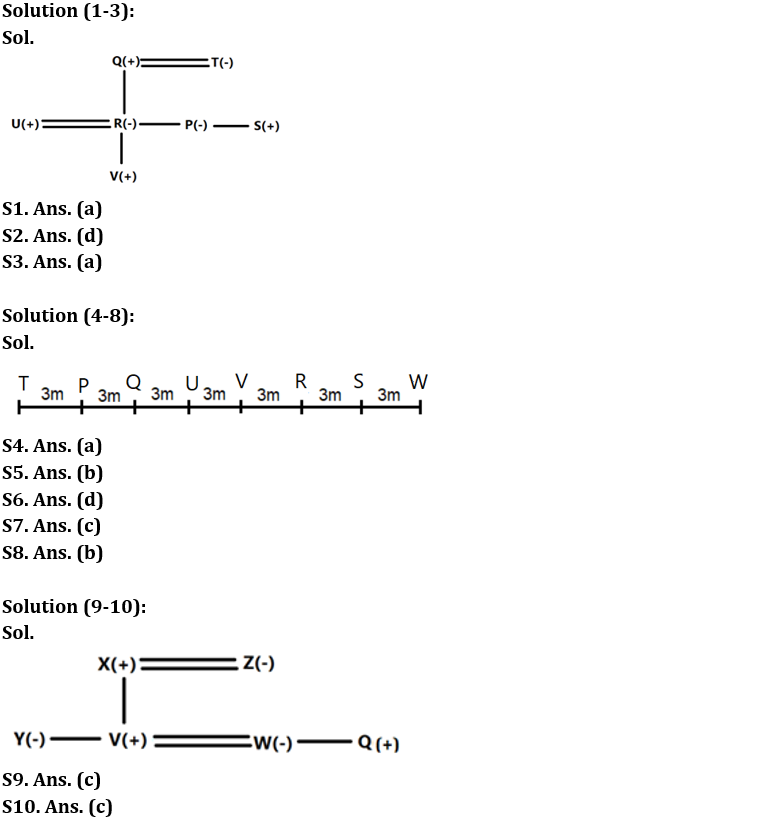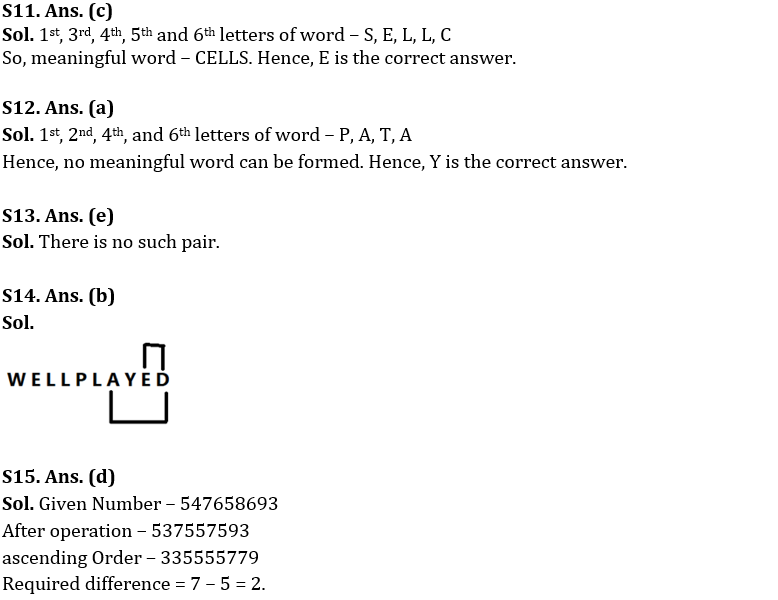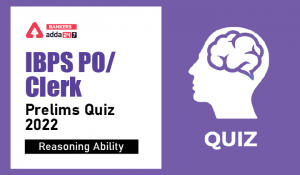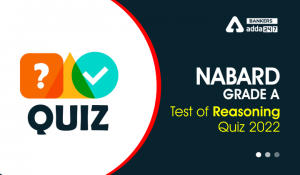Latest Banking jobs   »

# Reasoning Ability Quiz For IBPS RRB PO Clerk Prelims 2022- 12th July

Directions (1-3): Answer the questions based on the information given below.
There are seven members P, Q, R, S, T, U and V in a family which consists of three generations. There are only two married couples and only married couples have children. Male members are more than female members. U is the son in law of Q. T is the grandmother of V. Q has three children. S has two sisters. P is not the mother of V.

Q1. Who among the following are married couple?
(a) Q and T
(b) Q and P
(c) P and U
(d) S and U
(e) T and S

Q2. How is T related to U?
(a) Sister
(b) Mother
(c) Aunt
(d) Mother-in-law
(e) None of the above

Q3. How is P related to V?
(a) Aunt
(b) Uncle
(c) Father
(d) Sister
(e) None of the above

Directions (4-8): Answer the questions based on the information given below.
Eight persons P, Q. R, S, T, U, V and W sit in row facing towards north. The distance between adjacent persons is 3m. Q is 9m to the left of R. W sits at extreme right end. S is not 3m away from Q. Q does not sit at any extreme end. The distance between S and V is at most 6m. T sits immediate left of P who is not adjacent to R. S doesn’t sit to the left of R.

Q4. Who sits immediate left of Q?
(a) P
(b) W
(c) U
(d) V
(e) None of these

Q5. What is the distance between Q and W?
(a) 14m
(b) 15m
(c) 12m
(d) 9m
(e) None of these

Q6. Who among the following sits immediate right of R?
(a) W
(b) Q
(c) V
(d) S
(e) None of these

Q7. How many persons sit between P and S?
(a) Two
(b) Three
(c) Four
(d) One
(e) More than four

Q8. Who among the following sits 2nd to the left of the one who sits 5th from the left end?
(a) P
(b) Q
(c) T
(d) R
(e) S

Directions (9-10): Answer the questions based on the information given below.
Q is the brother-in-law of V. W is the daughter-in-law of X. Y and V are siblings. Y is not a male and not married. Z is the mother of V who is married to W.

Q9. Who is the father of Y?
(a) Q
(b) Z
(c) X
(d) Can’t be determined
(e) None of these

Q10. How many males are there in the family?
(a) One
(b) Two
(c) Three
(d) Four
(e) None of these

Q11. If it is possible to make a meaningful word from 1st, 3rd, 4th, 5th and 6th letters of word “SPELLCHECKER“, then which will be the 2nd letter of the word? If no such word be formed, mark the answer as ‘Y’. If more than one word can be formed mark the answer as ‘X’.
(a) Y
(b) L
(c) E
(d) C
(e) X

Q12. If it is possible to make a meaningful word from 1st, 2nd, 4th, and 6th letters of word “PARTIALLY“, then which will be the 3rd letter of the word? If no such word be formed, mark the answer as ‘Y’. If more than one word can be formed mark the answer as ‘X’.
(a) Y
(b) P
(c) A
(d) T
(e) X

Q13. How many pairs of letters are there in the word “ANAGRAMS” which has as many letters between them in the word as in the alphabetical series (Both forward and backward direction)?
(a) One
(b) Two
(c) Three
(d) Four
(e) None

Q14. How many pairs of letters are there in the word “WELLPLAYED” which has as many letters between them in the word as in the alphabetical series (Both forward and backward direction)?
(a) One
(b) Two
(c) Three
(d) Four
(e) None

Q15. If all the even digits of the number “547658693” are decreased by ‘1’ and then all the digits are arranged in ascending order from left to right, then what will be the difference between fourth digit from left end and second digit from the right end?
(a) 3
(b) 4
(c) 6
(d) 2
(d) None of these

Solutions#### Congratulations!Incorrect details? Fill the form again here

•Reasoning Ability Quiz For IBPS Clerk/PO...
•Reasoning Ability Quiz For IBPS RRB PO C...
•Reasoning Ability Quiz For NABARD Grade ...
•Reasoning Ability Quiz For IBPS Clerk Pr...
•Reasoning Ability Quiz For IBPS RRB PO C...
•Reasoning Ability Quiz For IBPS RRB PO C...